FV series FAQWhat is the relationship between the operation frequency range and voltage output of the FV-5300 F/V converter?The settings and the meanings of input range, output range, factor, and monitor display are as follows.

Input range Set the maximum value to be measured (maximum value of the monitor display) For instance, set a maximum measuring values such as 500 r/min and 100 m/min. Set the maximum values of output voltages and output currents with % Voltage: 0 to 10 V/0 to 100% Current output (optional): 0 to 16 mA/0 to 100 % or 4 mA to 20 mA/0 to 100% Coefficient for unit conversion. Use this information for converting a frequency to a unit of revolution (r/min) or speed (m/min). The value converted by dividing a frequency of an input signal by a factor is displayed.

The following formula can be established.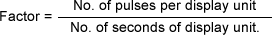(1)

The factor can be obtained from the following formula.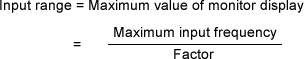(2)

The following diagram shows the relationship between input frequency and output voltage.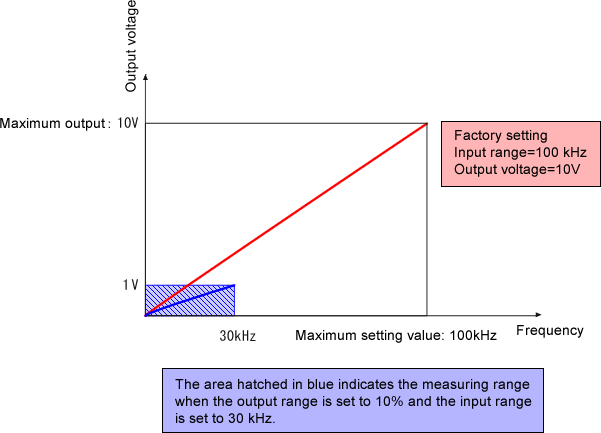Example 1: Setting the input range in the unit of revolution speed <r/min>.

Measurement condition Setting value

RP series Rotary Encoder: 360 P/R
Maximum revolution speed for measurement: 500 r/min
Output voltage: 0 to 1V/0 to 5000 r/min
Setting the input range in revolution r/min

Input range: 5.00k
Factor: 6
Output range: 10%
Measuring range: Monitor display 0 to 5.00k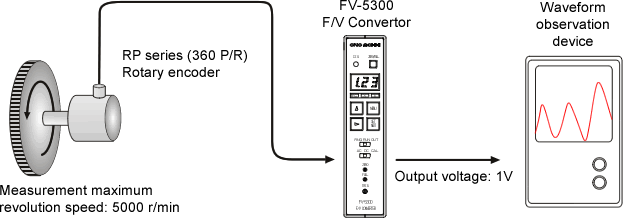1. How to use formula (1)

Factor

Obtain the factor from formula (1). The display units will be r (1 revolution) and min (minute) based on the measuring unit r/min. Based on the settings of pulse count per 1r=360 and number of seconds for display unit: 1 min=60s, the following formula is established.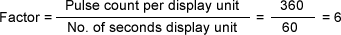Monitor display

Based on the setting of factor=6, the monitor display value is displayed in the unit of r/min automatically. 3-digit value is displayed. Use the information as a simple monitor.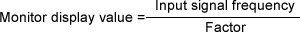Input range

Although the maximum measuring revolution is 5000 based on the condition, set 5.00k (k=factor of 1000) since the value comprises 3 digits. When a signal exceeding this setting value is input, Input Over occurs. In this case, it is necessary to review the condition such as increase of the input range setting value. A limit is set for the input range setting and when the setting exceeds the limit, an error message is displayed. Refer to "5.1 Input range" of the Instruction Manual for the details.

Output range

Set a maximum output voltage for the output range. This function limits the maximum output voltage up to 1 V when a device of the connection destination such as a recorder applies 1 V input. Refer to the "Setting value" column of the table provided above for the summary of the values that are obtained.

2. How to use formula (2)

Based on maximum revolution for measurement=5000 r/min and pulse count of the rotary encoder= 360 P/R: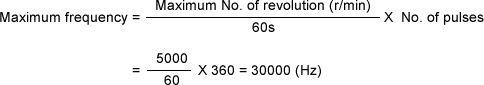Based on the measurement condition, input range=5000 and as a result of conversion of formula (3), the factor is: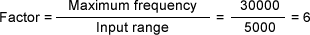The output range is the same as for item 1.

Example 2: Set an input range with frequency <Hz>

Measurement condition Setting value

RP series Rotary Encoder: 360 P/R
Maximum revolution speed for measurement: 5000 r/min Output voltage: 0 to 5V/0 to 5000 r/min
Setting the input range in frequency Hz

Input range: 30.0k (30,000)
Factor: 1
Output range: 50%
Measuring range: Monitor display 0 to 30.0k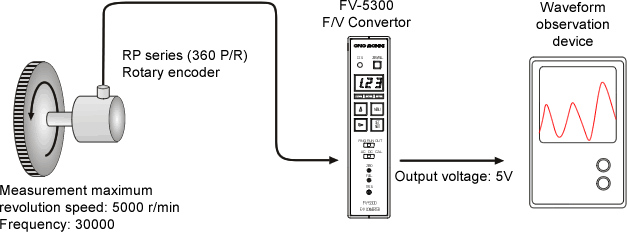Input range

Obtain the maximum frequency using the method 2 in Example 1. Based on the maximum revolution 5000 r/min;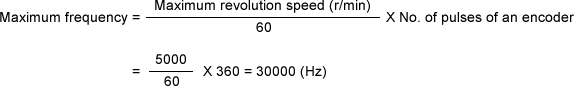Factor

Since the input range is set to the maximum frequency 30000, the factor is;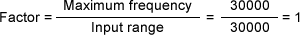Output range

Based on the measurement condition of 5 V, set the output range to 50%.
Refer to the "Setting value" column in the table shown above for the values that were obtained.

Example 3: Set an input range in speed (m/min).

Measurement condition Setting value

RP-721 roller encoder: 120 P/R
Roller diameter: πD = 0.2 m
Maximum measuring speed: 100 m/min
Output voltage: 0 to 10 V/0 to 100 m/min
Set the input range in speed m/min.

Input range: 100
Factor: 10
Output range: 100%
Measuring range: Monitor display 0 to 100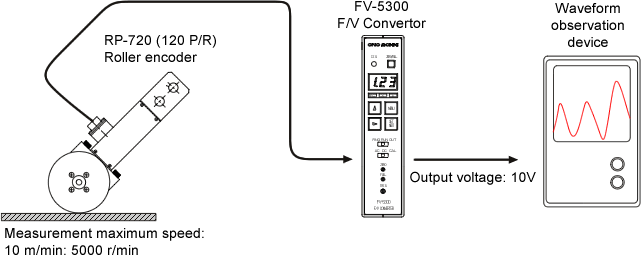Factor

Based on the specification of PR-721 in the measuring unit m/min;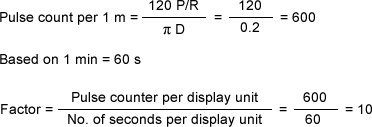Input range

Based on the maximum speed for measurement 100 m/min, set the input range to 100. When a signal exceeding this setting value is input, Input Over occurs. In this case, the setting needs to be reviewed.

Output range

Since the output voltage is 10 V based on the output condition, set 100%. Refer to the "Setting value" column of the table indicated above for the values that were obtained.

Revised:2011.09.20